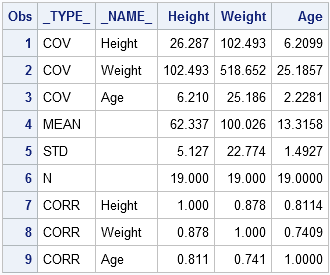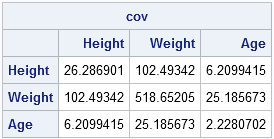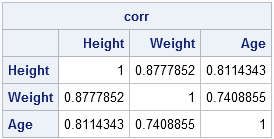Sample covariance matrices and correlation matrices are used frequently in multivariate statistics. This post shows how to compute these matrices in SAS and use them in a SAS/IML program. There are two ways to compute these matrices:

1. Compute the covariance and correlation with PROC CORR and read the results into PROC IML
2. Compute the matrices entirely with PROC IML

### Computing a covariance and correlation matrix with PROC CORR

You can use PROC CORR to compute the correlation matrix (or, more correctly, the "Pearson product-moment correlation matrix," since there are other measures of correlation which you can also compute with PROC CORR). The following statements compute the covariance matrix and the correlation matrix for the three numerical variables in the SASHELP.CLASS data set.

```ods select Cov PearsonCorr; proc corr data=sashelp.class noprob outp=OutCorr /** store results **/ nomiss /** listwise deletion of missing values **/ cov; /** include covariances **/ var Height Weight Age; run;```

The OutCorr data set contains various statistics about the data, as shown by running PROC PRINT:

`proc print data=OutCorr; run;`If you want to use the covariance or correlation matrix in PROC IML, you can read the appropriate values into a SAS/IML matrix by using a WHERE clause on the USE statement:

```proc iml; use OutCorr where(_TYPE_="COV"); read all var _NUM_ into cov[colname=varNames];   use OutCorr where(_TYPE_="CORR"); read all var _NUM_ into corr[colname=varNames]; close OutCorr;```

Notice that this SAS/IML code is independent of the number of variables in the data set.

### Computation of the covariance and correlation matrix in PROC IML

If the data are in SAS/IML vectors, you can compute the covariance and correlation matrices by using matrix multiplication to form the matrix that contains the corrected sum of squares of cross products (CSSCP).

Suppose you are given p SAS/IML vectors x1, x2, ..., xp. To form the covariance matrix for these data:

1. Use the horizontal concatenation operator to concatenate the vectors into a matrix whose columns are the vectors.
2. Center each vector by subtracting the sample mean.
3. Form the CSSCP matrix (also called the "X-prime-X matrix") by multiplying the matrix transpose and the matrix.
4. Divide by n-1 where n is the number of observations in the vectors.

This process assumes that there are no missing values in the data. Otherwise, it needs to be slightly amended. Formulas for various matrix quantities are given in the SAS/STAT User's Guide.

The following SAS/IML statements define a SAS/IML module that computes the sample covariance matrix of a data matrix. For this example the data are read from a SAS data set, so Step 1 (horizontal concatenation of vectors) is skipped. [Editor's Note 18AUG2011: In SAS 9.3 and beyond, use the built-in COV function.]

```proc iml; /** Prior to SAS 9.22, define module to compute a covariance matrix **/ start Cov(A); n = nrow(A); /** assume no missing values **/ C = A - A[:,]; /** subtract mean to center the data **/ return( (C` * C) / (n-1) ); finish;   /** read or enter data matrix into X **/ varNames = {"Height" "Weight" "Age"}; use sashelp.class; read all var varNames into X; close sashelp.class;   cov = Cov(X); print cov[c=varNames r=varNames];```Computing the Pearson correlation matrix requires the same steps, but also that the columns of the centered data matrix be scaled to have unit standard deviation. SAS/IML software already has a built-in CORR function, so it is not necessary to define a Corr module, but it is nevertheless instructive to see how such a module might be written. [Editor's Note 18AUG2011: In SAS 9.3 and beyond, use the built-in CORR function.]

```/** Prior to SAS 9.22, define module to compute a correlation matrix **/ start MyCorr(A); n = nrow(A); /** assume no missing values **/ C = A - A[:,]; /** center the data **/ stdCol = sqrt(C[##,] / (n-1)); /** std deviation of columns **/ stdC = C / stdCol; /** assume data are not constant **/ return( (stdC` * stdC) / (n-1) ); finish;   corr = MyCorr(X); print corr[c=varNames r=varNames];```You should use the built-in CORR function instead of the previous module, because the built-in function handles the case of constant data.

### Computation of the covariance and correlation matrix in PROC IML (post-9.2)

In November 2010, SAS released the 9.22 (pronounced "nine point twenty-two") release of SAS/IML software. This release includes the following:

• a built-in COV function which handles missing values in either of two ways
• new features for the built-in CORR function including
• handling missing values in either of two ways
• support for different measures of correlation, including rank-based correlations
ShareDistinguished Researcher in Computational Statistics

Rick Wicklin, PhD, is a distinguished researcher in computational statistics at SAS and is a principal developer of SAS/IML software. His areas of expertise include computational statistics, simulation, statistical graphics, and modern methods in statistical data analysis. Rick is author of the books Statistical Programming with SAS/IML Software and Simulating Data with SAS.

1.Dr.Wicklin,

How to handle missing value? My dataset has 37 obs, if I delete missing value, then only 29 obs left. So I have to use correlation of 29 obs to do sampling.
My SAS is 9.2 so can't use cov and corr function directly.

Thanks

•You can use PROC CORR to compute the matrix of correlations between pairs of variables. The missing values are still dropped, but only for the pairs of variables involved in computing each cell of the matrix.

By the way, SAS 9.2 is from 2008. You are missing tons of great features.